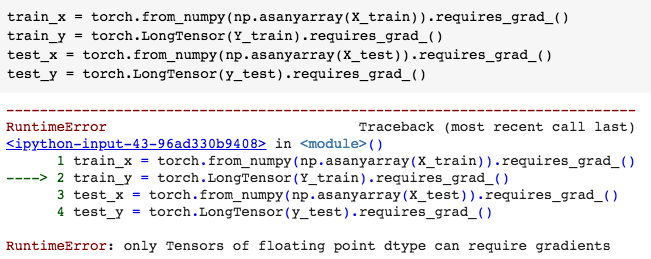# Multi-class classification variable set up (torch)

I’d like to prepare variables to run a multi class classification.

Note I try replicating the model shown in this post, where @Oli , @tymokvo , @god_sp33d and @Rojin contributed.

I have 10 variables split as (X_train,X_test) and 1 target variable split as (Y_train, Y_test).

My target/label is a 3 class (1,2,3)

I thought I would prepare them as follows:

``````> X_train = np.array(X_train, dtype=np.float32)
> X_test = np.array(X_test, dtype=np.float32)
> Y_train = np.array(Y_train, dtype=np.float32)
> y_test = np.array(y_test, dtype=np.float32)
``````

and then convert them to tensor as follows:

``````train_x = torch.from_numpy(np.asanyarray(X_train)).requires_grad_()
``````

unfortunately, when running code above I get as result:I would be very grateful if any one could shed some light on this.

Sincerely,

Remove long function

Thanks @kabilan , now it shows the following error:Hello Kabilan!

No, this isn’t correct. First, calling the `.long()` method on a
`LongTensor` is a no-op (does nothing). Second, the `train_y`
are to be used as categorical class labels, so they have to be
a `LongTensor`.

Best.

K. Frank

Hi Josep!

First, could you clarify what version of pytorch you are using?

What type of data structure are your `X_train`, `Y_train`, etc.,
before you wrap them in numpy arrays?

Just to clean things up a little, I would (try to) convert directly
from your original `X_train`, etc., to pytorch tensors.

(Please, in general, post text rather than screen-shots. It can then
be searched and copy-pasted.)

The main issue is that you neither need nor want gradients for

model so that they do a better job classifying your inputs. The
whole idea behind pytorch’s autograd facility is that it calculates
for you the gradients of your loss function with respect to your
model parameters so that you can use an optimization algorithm
such as gradient descent to “optimize” (change) those parameters
so that your model produces a lower loss function.

You don’t change the data you are training (or testing) with.

parameters will automatically be flagged with
`requires_grad = True`.

Note, a `FloatTensor` is (approximately) continuous, so it makes
sense to “do calculus” on it, i.e., calculate gradients.

But a `LongTensor` is made up of discrete (long) integers, so it
isn’t natural to “do calculus” on it, so pytorch doesn’t support
`requires_grad = True` for `LongTensor`s.

You should make your `train_x` and `test_x` “ordinary”
`FloatTensor`s (that will default to `requires_grad = False`),
and your `train_y` and `test_y` `LongTensors` (without
`.requires_grad_()`) (that pytorch will force to have
`requires_grad = False`).

Perhaps it would make sense to write a complete, runnable
script that generates some toy (random, if you choose) data,
packages that data in the appropriate pytorch tensors,
build a simple (one or two-layer) model, and pass one
batch of your input data through the model.

If you have trouble getting that to run, print out the types and
shapes of some of your tensors along the way, and post the
complete, runnable script, along with the output, and tell us
what problems you are having.

Good luck.

K. Frank

1 Like

@KFrank, it works!

Thank you very much for your time and explanation.

From now onwards, following your advice I shall post text rather than screen-shots.

1 Like